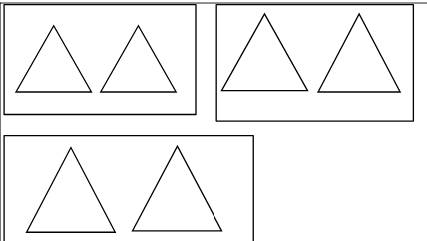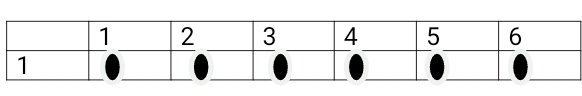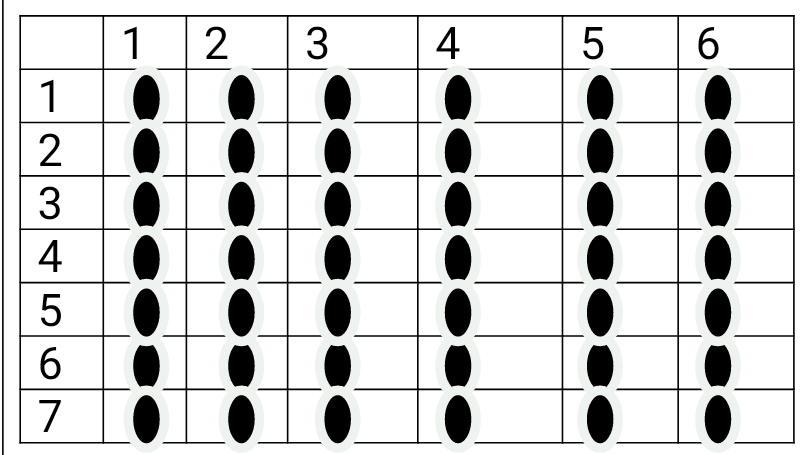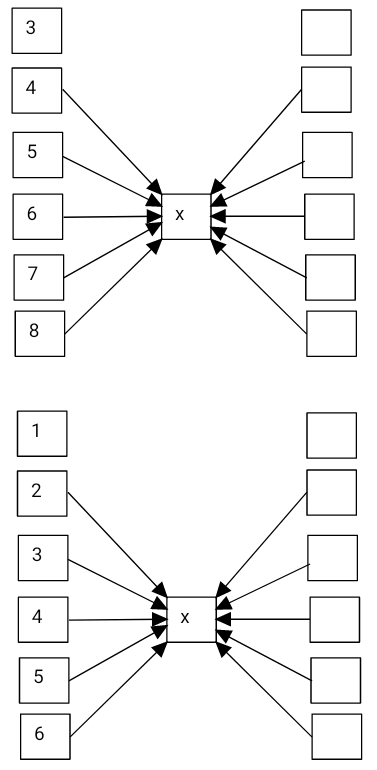# Lesson Notes By Weeks and Term - Primary 3

Multiplication

TERM: 1st Term

WEEK: 11

CLASS: Primary 3

AGE: 8 years

DURATION: 5 periods of 40 minutes each

DATE:

SUBJECT: Mathematics

TOPIC: Multiplication

SPECIFIC OBJECTIVES: At the end of the lesson, the pupils should be able to

1. Learn multiplication tables up to 5 times table

INSTRUCTIONAL TECHNIQUES: Explanation, question and answer, demonstration, practical, assessments

INSTRUCTIONAL MATERIALS: Multiplication tables, enlarged multiplication tables for the teacher, multiplication cards, videos from source-

PERIOD 1: Multiplication tables up to 5

PRESENTATION

TEACHER’S ACTIVITY

PUPIL’S ACTIVITY

STEP 1

MENTAL MATHS

The teacher begins the lesson with some mental calculations

Count forwards in 2s up to 20, in 3s up to 30, in 4s up to 40, in 5s up to 50

Pupils respond and participate

STEP 2

CONCEPT

DEVELOPMENT

The teacher draws three boxes on the board and draws two triangles in each boxThe teacher asks how many triangles are there altogether. And asks the learners to write the number sentence and answer in their  classwork book. (3 × 2 = 6 or 2 + 2 + 2 = 6, 6 triangles)

N.B: If no one writes 3 × 2 = 6, remind learners of the multiplication sign which represents 3 shelves/groups of 2.

The teacher asks: What the 3 means in 3 × 2 = 6? (The 3 tells us the number of groups.) and What the 2 means in 3 × 2 = 6? (The 2 tells us the number of triangles in one shelf/in one group.)

The teacher then tells the learners that calculations like 3 × 2 = 6 are multiplication calculations.

In pairs,

Pairs of learners are given the multiplication table.

The learners read the 1 times table, i.e. 1 × 1 = 1, 2 × 1 = 2, 3 × 1 = 3 … 9 × 1 = 9.

The learners read the 2 times table, i.e. 1 × 2 = 2, 2 × 2 = 4, 3 × 2 = 6 … 9 × 2 = 18

The same thing is done up to 5 times table.

The learners count forward in 1s up to 10, 2s up to 20, 3s up to 30, 4s up to 40, 5s up to 50.

The learners are then asked questions like:

a. how many 2s in 14?

b. how many 3s in 24?

c. What is common between the numbers in the 2 times table and the numbers that appear when you count in 2s? (The numbers are the same – if you count in 2s, you are counting the multiples of 2.)

The teacher Asks the same question for the other multiples, of 3, 4 and 5.

Pupils pay attention and participate

STEP 3

CLASS-WORK

The learners are given a set of multiplication cards (for the 1 to 5 times tables).

In this lesson, the learners should cut up the cards. They should write the answers on the back of each card, to prepare them for use in this and many other lessons.

Make sure that they

write the correct answers on the backs of the cards!

In this classwork session, learners will first cut and prepare the cards. Then they will do an activity in which they order the cards. After that they will play a multiplication card game using the cards, in pairs.

Rules of the game

1 One learner picks up a card (of the 1 to 5 multiplication cards) at a time and reads

it out loud.

2 The other learner gives the answer.

of the card each time if necessary.

1 Learners arrange the multiplication cards in order for the following times tables:

a × 1

b × 2

c × 3

d × 4

e × 5

2 Play the 1 to 5 multiplication card game. The teacher will explain the rules.

Pupils attempt their class work

STEP 4

HOME-WORK

Complete the table

 Multiplication Repeated addition Answer 1 4 x 3 3 + 3 + 3 + 3 2 5 x 4 4 + 4 + 4 + 4 + 4 3 2 + 2 + 2 + 2 8 4 5 x 3

Pupils attempt their class work

STEP 5

SUMMARY

The teacher summarizes by reminding the pupils how to solve multiplication tables up to the 5 times table.

She marks their class works, makes corrections where necessary and commends them positively

PERIOD 2: Multiplication using array diagrams

PRESENTATION

TEACHER’S ACTIVITY

PUPIL’S ACTIVITY

STEP 1

MENTAL MATHS

The teacher begins the lesson with some mental calculations

Calculate

1. 4 x 3 =

2. 5 x 1 =

3. 7 x 4 =

4. 3 x 2 =

5. 2 x 5 =

6. 6 x 3 =

7. 7 x 5 =

8. 8 x 2 =

9. 6 x 4 =

10. 5 x 3 =

Pupils respond and participate

STEP 2

CONCEPT

DEVELOPMENT

The teacher gives each learner an array diagram and puts an enlarged array diagram on the board.

The teacher then writes the following multiplication number sentence on the board:

3 × 4 =

and asks learners to use the array diagram to help them solve the problem.

The teacher asks how they used the array diagram to find the answer. (she Hides the diagram to show only the four columns and three rows.)

A learner is selected to come up to the board and explain to the class how they solved the problem.

The learners record the number sentence and answer in their classwork books.

The teacher explains that the right way of using the array diagram is as follows.

a. Hide away some of the columns by holding a book/paper. Then, hide away some of the rows, using a second piece of paper/book (2).

Paper 1 allows 4 dots in a row to be seen. This means we are showing the

4 x table.

Paper 2 can be moved up and down, to show a different multiple of 4, depending on how many rows are shown.

In this case, the diagram shows 3 × 4 = 12. (It is not 4 × 3. This would be shown by 4 rows with 3 dots in each row.)

Repeat the above steps using the array to show:

a. 4 × 4,

b. 5 × 4

c. 6 × 4

d. 7 × 4

e. 8 × 4

f. 9 × 4

The teacher helps the learners to see that they can find the answers by counting in 4s.

NOTE: As the number of groups (represented by the rows) increases, so the answer increases by 4 each time. The array diagram should always be used in the way explained above, i.e. by hiding unnecessary parts with paper.

CLASS ACTIVITY

In pairs

Each pair of learners are given two array diagrams while the teacher puts an enlarged array diagram on the board.

The teacher then writes the following multiplication number sentence on the board:

4 × 9 =

The learners are asked to use the array diagram to help them solve the problem.

A learner is selected to come up to the board and explain to the class how they solved the problem. The learners are encouraged to verbalise that the number on the left (i.e. 4) counts the number

of rows while the number on the right (i.e. 9) counts the number of columns (or the number of dots in a row).

The teacher asks one of the learners (from each pair) to move the papers so that they now show the product  9 × 4. (They should show 9 rows with 4 dots in each row.)

What do you see in the array diagrams of 4 × 9 and 9 × 4? (They both have the

same number of dots. I know 9 × 4 = 36, I can use this knowledge to answer 4 × 9).

The teacher puts another enlarged array diagram on the board to show 9 × 4 and discuss the similarities and differences between the two arrays with the class. (Same number of dots, number of dots in the rows and columns are interchanged.)

The whole process is repeated for

a. 5 × 6 with 6 × 5

b. 2 × 7 with 7 × 2.

The teacher gives some more examples of multiplications (e.g. 3 × 8, 7 × 6) if the learners work through

the activity quickly.

Pupils pay attention and participate

STEP 3

CLASS-WORK

The learners are given a set of multiplication cards. The cards should have been cut up and prepared in the previous lesson so that they can be arranged in order.

Rules of the game

1 One learner picks up a card (of the 1 to 5 multiplication cards) at a time and reads

it out loud.

2 The other learner gives the answer.

3 Learners take turns to ask and answer questions, checking the answers at the back of the card each time if necessary.

1 Learners arrange the multiplication cards in order for the following times tables:

a × 1

b × 2

c × 3

d × 4

e × 5

2 Play the 1 to 5 multiplication card game. Your teacher will explain the rules.

Pupils attempt their class work

STEP 4

HOME-WORK

Complete the table

 Multiplication Repeated addition Answer 1 6 x 4 4 + 4 + 4 + 4 + 4 + 4 2 6 + 6 + 6 + 6 3 5 + 5 + 5 + 5 + 5 + 5 + 5 + 5 + 5 4 5 x 9

Pupils attempt their class work

STEP 5

SUMMARY

The teacher summarizes by reminding the pupils how to represent multiplication pictorially using an array diagram.

She marks their class works, makes corrections where necessary and commends them positively

PERIOD 3: The 6 times table

PRESENTATION

TEACHER’S ACTIVITY

PUPIL’S ACTIVITY

STEP 1

MENTAL MATHS

The teacher begins the lesson with some mental calculations

Calculate

a. 6 + 6 =

b. 18 + 6 =

c. 36 + 6 =

d. 48 + 6 =

e. 30 + 6 =

f. 54 + 6 =

g. 12 + 6 =

h. 60 + 6 =

i. 24 + 6 =

j. 42 + 6 =

Pupils respond and participate

STEP 2

CONCEPT

DEVELOPMENT

The teacher gives each pair of learners an array diagram. The teacher writes the following multiplication number sentence on the board:

1 × 6 =

The teacher asks learners to use the array diagram to help them solve the problem, by hiding unnecessary rows and columns in the array.

The teacher encourages learners to verbalise that they identified 1 row with 6 dots in the row in the

array. The teachers helps the learners to demonstrate this as shown below:The learners records the number sentence and answer in their class work books. The learners repeat the above steps using the array to find the value of 2 × 6 and 3 × 6 and records the answers in their classwork books.

The teacher asks how many should we add to 3 × 6 to get the answer for 4 × 6? (6 because there

are 6 dots in a row, we are working out the multiples of 6.)

What do you notice about the answers that you recorded in your classwork book? (Learners may say that they see that the answers increase by 6 each time). The teacher shows learners the array diagrams for each of the calculations (as shown

below) to help learners see that each time there is a row of 6 added.The teacher helps the learners to understand that 6 is added each time by writing out the repeated addition sum for each diagram on the board:

6 + 6 is 12

6 + 6 + 6 is 18

6 + 6 + 6 + 6 is 24

CLASS ACTIVITY

The teacher asks pairs of learners to use their array diagrams to find the answers to the following:

5 × 6

6 × 6

7 × 6

8 × 6

9 × 6

Learners need to record the number sentences and answers in their classwork books.

The teacher asks How can we find the answer for each of them? (By adding 6 to the

Learners can then use these calculations and answers to create the rest of the 6 times

table (up to 9 × 6).

The teacher draws this table on the board:

The entire class completes the table together

 1 2 3 4 5 6 7 8 9 x 6 6 12 18 24 30 36 42 48 54

A learner is asked to represent the table as a flow chart on the board

Pupils pay attention and participate

STEP 3

CLASS-WORK

Each pair or each group of learners is given a set of multiplication cards for the 6 times table. Learners need to prepare these cards (cut them up and write the answers on the back) as they did for the multiplication cards of the 1-5 times tables. There

are 4 activities suggested below – Select 2 activities to do in this lesson. Keep the cards safe since they will be used in several lessons this term.

(You should also vary the activities that

you choose to use so that the learners don’t get bored doing the same activities each time)

Rules of the games

1 Learners work alone.

a Learners shuffle the cards.

b Learners take a number sentence card.

c Learners need to say the answer to the number sentence shown on each card to

themselves.

d Learners check the answers by looking at the back of the card.

2 Learners work in pairs.

a Learners shuffle the cards.

b One learner holds up a number sentence for the second learner to read.

c The second learner must read the number sentence and give the answer.

d Learners check the answers by looking at the back of the card.

e The second learner then holds up a number sentence card for the first learner.

f Keep going until all the cards have been read.

3 Learners work in groups of 3.

a Learners shuffle the cards.

b Learners lay out the cards with the answers facing up.

c One learner calls out a multiple – any one of the 1 to 9 times tables.

d The other two learners need to find the card with the answer to the

multiplication number sentence.

e The first learner to find the card gets to keep the card. The learner who has the

most cards at the end wins.

f When there are no more cards, the game can be played again with a different

learner calling out the multiplication questions.

4 Learners work alone.

a Learners shuffle the cards.

b Learners lay out the cards with the answers facing up.

c Learners think of a number sentence which matches with the answer facing up.

d Learners check the number sentence.

Pupils attempt their class work

STEP 4

HOME-WORK

Complete the table

 Multiplication Repeated addition Answer 1 3 x 6 6 + 6 + 6 2 4 x 6 3 6 + 6 + 6 + 6 + 6 4 6 x 6 5 6 + 6 + 6 + 6 + 6 + 6 + 6

Pupils attempt their class work

STEP 5

SUMMARY

The teacher summarizes by reminding the pupils how to solve the 6 times table.

She marks their class works, makes corrections where necessary and commends them positively

PERIOD 4: The 6 times table

PRESENTATION

TEACHER’S ACTIVITY

PUPIL’S ACTIVITY

STEP 1

MENTAL MATHS

The teacher begins the lesson with some mental calculations

Calculate

a. 6 x 1 =

b. 6 x 2 =

c. 6 x 3 =

d. 6 x 4 =

e. 6 x 5 =

f.  6 x 3 =

g. 6 x 2 =

h. 6 x 1 =

I. 6 x 5 =

j. 6 x 4 =

Pupils respond and participate

STEP 2

CONCEPT

DEVELOPMENT

The teacher gives pairs of learners a set of multiplication cards for the 6 times table and asks the learners to put the cards in order on their desks.

In pairs, learners hold up each card from the 6 times table in order to read it out loud to their partner. Learners take turns to hold up the cards in order for the other learner to read the number sentence aloud and give the answers. Learners check the answers each time.

CLASS ACTIVITY

Write the following word problem on the board:

There are 4 packets

of 6 eggs each.

How many eggs are there in total?

(The word problem must be written on three lines as shown above to assist learners to identify the critical information/numbers needed to solve the problem)

The teacher reads the problem and lets the learners read the problem until they read it fluently.

The teacher then underlines the numbers (4 and 6) and also underlines the question (How many eggs are there in total?) with a wavy line.

The teacher tells the learners draw four groups of circles, with 6 in each group as shown below.NOTE: It is important that learners are aware of the size of the group in multiplication (i.e. 6 in this question).

The teacher lets learners write the number sentence present their number sentence and determine the operation.

The learners are also allowed to solve the number sentence (4 × 6 = 24).

The teacher then asks the learners

What is the answer for the word problem? (There are 24 eggs.)

The Learners must answer with the unit, 24 eggs.

CLASS ACTIVITY

The teacher lets the learners solve the problem below by following the same steps as in Activity 2.

The teacher gives the learners array diagrams to work with.

There are 7 plastic bags

that can hold 6 mangoes each.

How many mangoes are there in total?

(The only difference between activity 1 and activity 2 is that learners use an array diagram in activity 2 instead of drawing circles)

The array diagram for this problem is as follows.The number sentence is 7 × 6 = 42.

Pupils pay attention and participate

STEP 3

CLASS-WORK

Each pair or each group of learners is given a set of multiplication cards for the 6 times table. Learners need to prepare these cards (cut them up and write the answers on the back) as they did for the multiplication cards of the 1-5 times tables. There

are 4 activities suggested below – Select 2 activities to do in this lesson. Keep the cards safe since they will be used in several lessons this term.

(You should also vary the activities that

you choose to use so that the learners don’t get bored doing the same activities each time)

Rules of the game

1 Learners work alone.

a Learners shuffle the cards.

b Learners take a number sentence card.

c Learners need to say the answer to the number sentence shown on each card to themselves.

d Learners check the answers by looking at the back of the card.

2 Learners work in pairs.

a Learners shuffle the cards.

b One learner holds up a number sentence for the second learner to read.

c The second learner must read the number sentence and give the answer.

d Learners check the answers by looking at the back of the card.

e The second learner then holds up a number sentence card for the first learner.

f Keep going until all the cards have been read.

3 Learners work in groups of 3.

a Learners shuffle the cards.

b Learners lay out the cards with the answers facing up.

c One learner calls out a multiple – any one of the 1 to 9 times tables.

d The other two learners need to find the card with the answer to the multiplication number sentence.

e The first learner to find the card gets to keep the card. The learner who has the most cards at the end wins.

f When there are no more cards, the game can be played again with a different learner calling out the multiplication questions.

4 Learners work alone.

a Learners shuffle the cards.

b Learners lay out the cards with the answers facing up.

c Learners provide a number sentence which matches with the answer facing up.

d Learners check that they gave a correct number sentence.

Pupils attempt their class work

STEP 4

HOME-WORK

Complete the table

 Multiplication Repeated addition Answer 1 9 x 6 6 + 6 + 6 + 6 + 6 + 6 + 6 + 6 + 6 2 8 x 6 3 6 + 6 4 3 x 6 5 6 + 6 + 6 + 6

Pupils attempt their class work

STEP 5

SUMMARY

The teacher summarizes by reminding the pupils how to solve the 6 times table.

She marks their class works, makes corrections where necessary and commends them positively

PERIOD 5: Weekly Test/consolidations

TEACHER’S ACTIVITY: The teacher revises all the concepts treated from period 1-4 and gives the pupils follow through exercises, quiz and tests . She marks the exercises, makes corrections and commends the pupils positively.

PUPIL’S ACTIVITY: The pupils work on the worksheets and exercises given by the teacher individually

Today we are going over what we learned this week. We are learning more about multiplication.

NOTE: Question 3 of the activity calls for the learners to play the multiplication card game.

Learners need the cards for the 5 and 6 times tables for the game today.

Rules of the game

Learners work in pairs.

a Learners shuffle the cards.

b Learners lay out the cards with the answers facing up.

c The first learner selects a card for the second learner.

d The second learner must say whether the answer is part of the 5 or 6 times table.

e The second learner must also say either the number that comes before the answer

shown, or the number that comes after the number shown.

f For example: If the number 12 is shown, the second learner will say “It is from the 6 times table and the number before it is 6.”

g The second learner then selects a card for the first learner, and the game proceeds in the same way.

Complete the table

 Multiplication Repeated addition Answer 1 2 x 5 5 + 5 2 3 x 5 3 5 + 5 + 5 + 5 4 5 x 5 5 5 + 5 + 5 + 5 + 5 +5 6 7 x 5 7 5 + 5 + 5 + 5 + 5 + 5 + 5 + 5 40 8 5 + 5 + 5 + 5 + 5 + 5 + 5 + 5 + 5 45

Complete the table

 Multiplication Repeated addition Answer 1 2 x 6 6 + 6 2 3 x 6 3 6 + 6 + 6 + 6 4 6 x 6 5 6 + 6 + 6 + 6 + 6 +6 6 7 x 6 7 6 + 6 + 6 + 6 + 6 + 6 + 6 + 6 48 8 6 + 6 + 6 + 6 + 6 + 6 + 6 + 6 + 6 54

Complete the flow diagrams